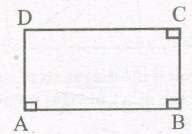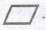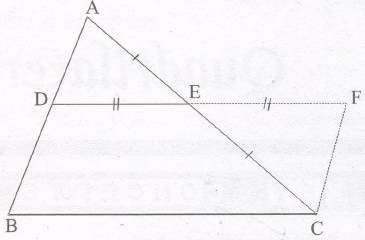## Quadrilaterals Notes For Class 9

A quadrilateral is a closed figure obtained by joining four point (with no three points collinear) in an order.

(i) Four vertices
(ii) Four sides
(iii) Four angles
(iv) Two diagonals

### SUM OF THE ANGLES OF A QUADRILATERAL

Statement: The sum of the angles ofa quadrilateral is 360°

1. Trapezium : It is quadrilateral in which one pair of opposite sides are parallel.

2. Parallelogram : It is a quadrilateral in which both the pairs of opposite sides are parallel.

3. Rectangle : It is a quadrilateral whose each angle is 90°. ABCD is a rectangle.
(i) ∠A+ ∠B = 90° + 90° = 180° ⇔ AD || BC
(ii) ∠B+ ∠C= 900 + 900 = 180° ⇔ AB || DCRectangle ABCD is a parallelogram also.

4. Rhombus : It is a quadrilateral whose all the sides are equal.

5. Square : It is a quadrilateral whose all the sides are equal and each angle is 90°.

6. Kite : It is a quadrilateral in which two pairs of adjacent sides are equal.

Note :
• Square, rectangle and rhombus are all parallelograms.
•Kite and trapezium are not parallelograms.
• A square is a rectangle.
• A square is a rhombus
• A parallelogram is a trapezium.

#### PARALLELOGRAM:

A parallelogram is a quadrilateral in which opposite sides are parallel. It is denoted by### PROPERTIES OF PARALLELOGRAM:

1. A diagonal of a parallelogram divides it into two congruent triangles.

2. The opposite sides of a parallelogram are equal.

Theorem : If each pair of opposite sides of a quadrilateral is equal, then it is a parallelogram.

3. The opposite angles of a parallelogram are equal.

Theorem : If in a quadrilateral, each pair of opposite angles is equal, then it is a parallelogram.

4. The diagonals of a parallelogram bisect each other.

Theorem :
If the diagonals of a quadrilateral bisect each other, then it is a parallelogram.

#### MID POINT THEOREM (BASIC

PROPORTIONALITY THEOREM)

Statement 1:

The line segment joining the mid-points of any two sides of a triangle is parallel to the third sideStatement 2:

The line drawn through the mid-point of one side of a triangle, parallel to another side bisects the third side.

### NCERT Books Free Pdf Download for Class 5, 6, 7, 8, 9, 10 , 11, 12 Hindi and English Medium

 Mathematics Biology Psychology Chemistry English Economics Sociology Hindi Business Studies Geography Science Political Science Statistics Physics Accountancy